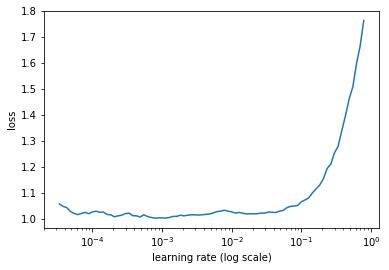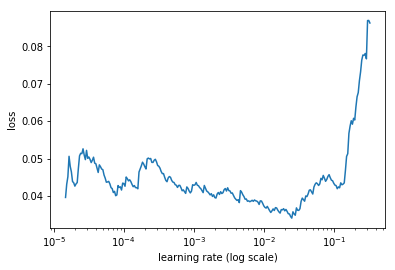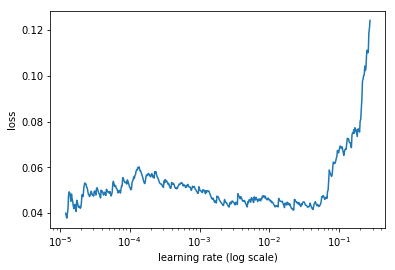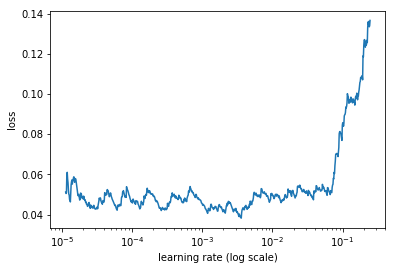# Loss-learning curve: what does this tell about my dataDoes this mean that my data is not good enough for any learning to take place?

The finder probably did the entire epoch without attaining a sufficient drop for the loss, then stopped. There is some work in progress to fix this.

In any case, try to reduce the batch size and run the finder again.

Should you get the same dismal result, make the following provisional modification:

In `learner.py`, locate the function `lr_find(...)`, delete it entirely and paste the following code in its place:

`````` def lr_find(self, start_lr=1e-5, end_lr=10, wds=None, linear=False, run_for=1):
"""Helps you find an optimal learning rate for a model.

It uses the technique developed in the 2015 paper
`Cyclical Learning Rates for Training Neural Networks`, where
we simply keep increasing the learning rate from a very small value,
until the loss starts decreasing.

Args:
start_lr (float/numpy array) : Passing in a numpy array allows you
to specify learning rates for a learner's layer_groups
end_lr (float) : The maximum learning rate to try.
wds (iterable/float)
run_for (Int) : the number of cycles we want to run the finder over.

Examples:
As training moves us closer to the optimal weights for a model,
the optimal learning rate will be smaller. We can take advantage of
that knowledge and provide lr_find() with a starting learning rate
1000x smaller than the model's current learning rate as such:

>> learn.lr_find(lr/1000)

>> lrs = np.array([ 1e-4, 1e-3, 1e-2 ])
>> learn.lr_find(lrs / 1000)

Notes:
lr_find() may finish before going through each batch of examples if
the loss decreases enough.

.. _Cyclical Learning Rates for Training Neural Networks:
http://arxiv.org/abs/1506.01186

"""
self.save('tmp')
layer_opt = self.get_layer_opt(start_lr, wds)
self.sched = LR_Finder(layer_opt, run_for*len(self.data.trn_dl), end_lr, linear=linear)
self.fit_gen(self.model, self.data, layer_opt, run_for)
``````

Now you can specify an additional param: `run_for=N`, by which the finder will run for N epochs. Do your experiments and report back. Don’t be afraid: you can revert to the original file just by doing a `git pull`.

I was getting a similar loss vs learning rate curve using a VGG16 architecture with a batch size of 128 on the dogs & cats dataset (size=224).I tried decreasing the batch size to a standard 64 and the new curve had a shape similar to the one before.Then I tried experimenting with the suggested lr_find(…) function with run_for parameter at 2 and 3. Here are the results for 2 runs:This one below is for 3 runs:Could someone please elaborate and explain this behavior?
Thanks a ton.